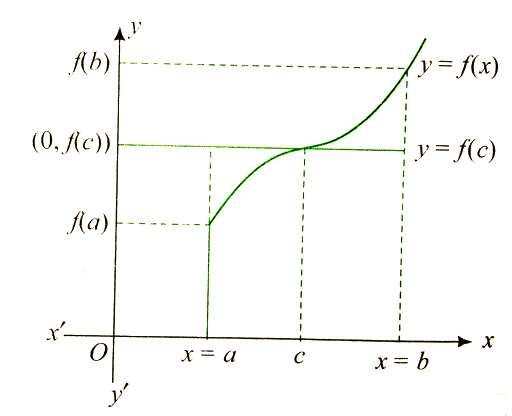Class 12 Math Calculus Area

If y=f(x) is a monotonic function in (a,b), then the area bounded by the ordinates at .Differentiating w.r.t. c, we get

For maxima and minima ,

Hence,

Hence, A is minimum when .
The value of the parameter a for which the area of the figure bounded by the abscissa axis, the graph of the function , and the straight lines, which are parallel to the axis of ordinates and cut the abscissa axis at the point of extremum of the function, which is the least, isConnecting you to a tutor in 60 seconds.
Similar topics
relations and functions
integrals
trigonometric functions
inverse trigonometric functions
application of derivativesConnecting you to a tutor in 60 seconds.